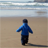# QlikView App Dev

Discussion Board for collaboration related to QlikView App Development.

Announcements
Now Live: Qlik Sense SaaS Simplified Authoring – Analytics Creation for Everyone: READ DETAILS
cancel
Showing results for
Did you mean:Partner - Contributor III

## Set analysis with NOT IN

Hello community,

I resume this post ETL with NOT IN | Qlik Community with some variations. (thanks again to Henric Cronström's Profile | Qlik Community).

‌I uploaded two tables linked by a field named %PDS_JOIN. Using Set Analysis I must to obtain the following result:

SELECT

TABLE_02.PDS,

SUM(TABLE_02.CAPACITA) AS CAPACITA

FROM TABLE_02

WHERE

(

TABLE_02.[DATA INIZIO MOV.]<=[@data1] AND

TABLE_02.[DATA FINE MOV.]>=[@data1]

OR

TABLE_02.[DATA INIZIO MOV.]<=[@data2] AND

TABLE_02.[DATA FINE MOV.]>=[@data2]

)

AND

TABLE_02.[DATA INIZIO FORN.]<=[@data1] AND

TABLE_02.[DATA FINE FORN.]>=[@data1]

AND

(

TABLE_02.%PDS_JOIN Not In (

SELECT TABLE_01.[%PDS_JOIN] FROM TABLE_01

WHERE TABLE_01.[DATA RIF.] between  [@data1] and [@data2]

)

OR

TABLE_02.%PDS_JOIN In (

SELECT TABLE_01.[%PDS_JOIN] FROM TABLE_01

WHERE TABLE_01.[DATA VAL.] between  [@data1] and [@data2]

)

)

GROUP BY TABLE_02.PDS

@Data1 and @Data2 are two values that the users choose using two Calendar object. My filters are:How can I write the expression in a pivot table to obtain the sum TABLE_02.CAPACITA ?

Thank you

1 Solution

Accepted SolutionsPartner - Contributor III
Author

Here's the answer to the problem: "Select not in" in Set Analysis

6 RepliesChampion

Simply write:  sum(CAPACITA ) as expressionPartner - Champion III

A small word of advise: do not try to translate SQL into QV expressions or QV Script code. You will get sub-optimal code with often very bad behavior and/or performance. Start from the business rule, or the actual requirement. Can you tell us what exactly you are trying to filter out?Partner - Contributor III
Author

Thank you Peter.

This query is used for a pivot with PDS and SUM(CAPACITA) in Business Object: I have two QVD files and my target is create the same pivot.

In my script I don't translate the query, but load TABLE1 and TABLE2 separated without WHERE or EXISTS conditions and only the field [%PDS_JOIN] creates the link in QV schema.

My question is: how can I write the SUM expression of CAPACITA using the set analysis?

Or am I doing something wrong in my approach?

(PS: am I in the correct section of the community?)Partner - Champion III

Well, if I understand you correctly you currently load all data, connect tables 1 and 2 through a single key field and end up with possibly too much data because there isn't any filtering being done in the script.

Let's assume that you want to use Set Analysis to do some filtering (if not, you wouldn't need Set Analysis and Balraj's solution would be perfect - selections will do the rest), then what has to be filtered out? What filter conditions do you want to apply in Set Analysis?

Peter

(PS: not really - this belongs more in "App Development", "Creating Analytics" or possibly "New To QlikView" if set analysis is completely new to you)Partner - Contributor III
Author

Exactly Peter, there is only a single key field named %PDS_JOIN.

Here's the situation:

@Data1 and @Data2 are two values that the users choose using two Calendar object. My filters are:

1 - on TABLE_02:

(

TABLE_02.[DATA INIZIO MOV.]<=[@data1] AND

TABLE_02.[DATA FINE MOV.]>=[@data1]

OR

TABLE_02.[DATA INIZIO MOV.]<=[@data2] AND

TABLE_02.[DATA FINE MOV.]>=[@data2]

)

AND

TABLE_02.[DATA INIZIO FORN.]<=[@data1] AND

TABLE_02.[DATA FINE FORN.]>=[@data1]

and

2 - on TABLE_01:

AND

(

TABLE_02.%PDS_JOIN Not In (

SELECT TABLE_01.[%PDS_JOIN] FROM TABLE_01

WHERE TABLE_01.[DATA RIF.] between  [@data1] and [@data2]

)

OR

TABLE_02.%PDS_JOIN In (

SELECT TABLE_01.[%PDS_JOIN] FROM TABLE_01

WHERE TABLE_01.[DATA VAL.] between  [@data1] and [@data2]

)

)

There are the syntax IN and NOT IN in the 2nd part.

How can I write the expression with Set Analysis in SUM(CAPACITA)?

(PS: I move my question in the correct section. Thank you.)Partner - Contributor III
Author

Here's the answer to the problem: "Select not in" in Set Analysis